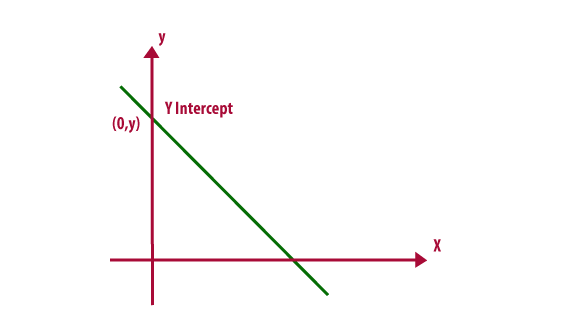# Y Intercept Formula

In analytic geometry, using the common convention that the horizontal axis represents a variable x and the vertical axis represents a variable y and a y-intercept is a point where the graph of a function or relation intersects with the y-axis of the coordinate system.The Y Intercept Formula of a line Ax + By = C is,

$\large y=\frac{C}{B}$

If, the Y Intercept Formula of a line in the slope-intercept form y = mx + c is,

$\large y=c$

Where,
m = slope of the line.
c = y-intercept of the line.

If, the Y Intercept Formula of a line in the point-slope form y – b = m(x – a) is,

$\large y=\; -am+b$

Where,
m is the slope of the line.
(a,b) is a point on the line.

### Solved Example

A solved problem on Y Intercept is given below:

Question 1: Find the y-intercept of the equation 3x + 4y = 12.

Solution:
To find the y-intercept, set x = 0 and solve for y.

3(0) + 4y = 12
0 + 4y = 12
4y = 12
y = 12/4
y = 3

Alternatively,

Comparing the given equation with Ax + By = C,

A = 3, B = 4, C = 12

y-intercept = C/B = 12/4 = 3

#### 1 Comment

1. Somnath

When we use the y intercept is equal to Zero and Y≠0 in the calculation formula?